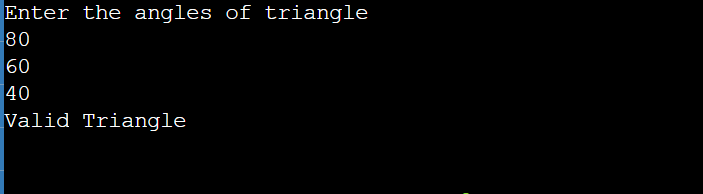# Bash Shell Script to Check whether triangle is valid or not if sides are given

• Last Updated : 05 Sep, 2022

In this article, we will discuss how to find out whether the given angles create a valid triangle or not. Here we will take input from the user and, based on the input, we will check whether the angles are equal, less than, or greater than 180 degrees and print the desired result.

### Example

```Inputs : 80 60 40
Output : Valid Triangle

Inputs : 55 44 30
Output : Invalid Triangle ```

A triangle is valid if the sum of all its angles is equal to 180 degrees.

### Steps

1. Take the user’s input of three angles as A1, A2, and A3.
• In Shell Script, “echo” is used to display the text/string on the console.
• “read” is used to read text from a console.
2. Add all of the angles together and store the result in a variable.
• Then the input is stored in the sum variable.
3. Then check if the sum of angles is equal to 180 degrees.
• To check whether the sum is equal to 180 degrees, we use “-eq”, which means equals
4. If true, print valid. Otherwise, the print is invalid.
• If the condition is satisfied, it prints “Valid Triangle”; else it prints “Invalid Triangle.”
• In the end, we have used “fi”, which indicates the end of the if loop.
```# Taking input from user
echo "Enter the angles of triangle"

# storing the sum of angles in sum variable
sum=\$((A1+A2+A3))

# checking if sum is equal to 180
if [ \$sum -eq 180 ]

# if condition is satisfied
then
echo "Valid triangle"

# if condition is not satified
else

echo "Invalid triangle"

# end of if loop
fi```

Output:output

Time complexity: O(1) as constant operations are done

Auxiliary space: O(1)

My Personal Notes arrow_drop_up